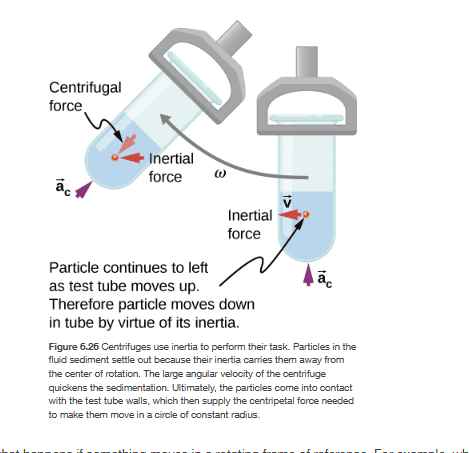# Centrifugal and inertial force in centrifuge

• ChiralSuperfields

#### ChiralSuperfields

Homework Statement
Would anybody please know why in my textbook below they show the centrifugal force with a inertial force? I though the centrifugal force was a result of the particle's inertia when viewed from an inertial frame. I don't understand why the diagram shows an inertial force when there was no previous mention of it in the textbook.

Many thanks!
Relevant Equations
## F_c = \frac {mv^2}{r} ## is a real force from an inertial frame.An inertial force is a force that appears in a non-inertial frame but not in an inertial frame. The centrifugal force is an example of an inertial force. Also, ##\frac{mv^2}{r}## is not a force in an inertial frame; it is mass × acceleration. It appearss the centrifugal force in a rotating frame. I don't understand the label "Inertial force" next to the centrifuge tube on the right.

•ChiralSuperfields
An inertial force is a force that appears in a non-inertial frame but not in an inertial frame. The centrifugal force is an example of an inertial force. Also, ##\frac{mv^2}{r}## is not a force in an inertial frame; it is mass × acceleration. It appearss the centrifugal force in a rotating frame. I don't understand the label "Inertial force" next to the centrifuge tube on the right.
Thank you for your help @kuruman ! Sorry what did you mean by
##\frac{mv^2}{r}## is not a force in an inertial frame; it is mass × acceleration.
?

I though ##\frac {mv^2}{r} ## is a force in an inertial frame since ##a_c = \frac {v^2}{r}## so ##F_c = ma_c##. Did you mean the centripetal force is not a force in a non-inertial frame?

Many thanks!

##\frac{mv^2}{r}## is not a force in an inertial frame;
It is an identifiable force, but it is not a specific applied force. The centripetal force is that component of the net force which is normal to the velocity.

•ChiralSuperfields
It is an identifiable force, but it is not a specific applied force.
For a mass rotating at the end of a taut string, ##F_{net}=ma## translates to ##T=\frac{mv^2}{r}##. The net force is on the LHS and mass times acceleration on the RHS. I see no benefit in thinking of the RHS as a force but I see harm in the confusion it might cause in the minds of students who are learning how to construct and interpret FBDs.

•ChiralSuperfields
What book is this? That arrow labeled ac does not make sense either. The plane of the circular trajectory is horizontal so the centripetal acceleration is horizontal. And so is the (inertial) centrifugal force.
And the tube is vertical when the centrifuge is not spinning so there is actually no centripetal acceleration or inertial force.

•ChiralSuperfields
What book is this? That arrow labeled ac does not make sense either. The plane of the circular trajectory is horizontal so the centripetal acceleration is horizontal. And so is the (inertial) centrifugal force.
And the tube is vertical when the centrifuge is not spinning so there is actually no centripetal acceleration or inertial force.
Thank you for your replies @haruspex , @kuruman and @nasu !

It is University Physics Volume 1 (Openstax)